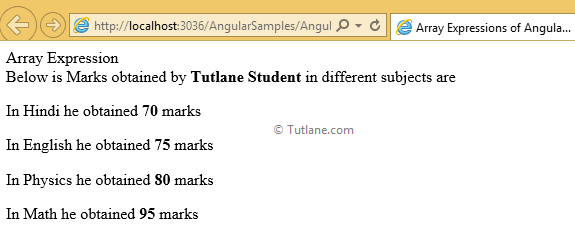Array Expressions in AngularJS with Example

Here we will learn what are the array expressions in angularjs and how to use array expressions in angularjs with example.

AngularJS Array Expressions

Array expressions in angularjs are the expression that hold an array and use those array objects while evaluating array expressions in angularjs.

Example of Array Expressions in AngularJS

<script type="text/javascript">

var app = angular.module('expressionApp', [])

app.controller("expressionController", function (\$scope) {

\$scope.name = " Tutlane Student";

\$scope.marks = [70, 75, 80, 95];

});

</script>

Write the following code under starting of body tag <body> for array expressions in angularjs like as shown below

<body ng-app="expressionApp">

<form id="form1">

<div ng-controller="expressionController">

<div>Array Expression</div>

Below is Marks obtained by <b>{{name}}</b> in different subjects are</br>

<p>In Hindi he obtained <b>{{marks}}</b> marks</p>

<p>In English he obtained <b>{{marks}}</b> marks</p>

<p>In Physics he obtained <b>{{marks}}</b> marks</p>

<p>In Math he obtained <b>{{marks}}</b> marks</p>

</div>

</form>

</body>

Following is the full example code for array expressions in angularjs with live demo as show below

<html xmlns="http://www.w3.org/1999/xhtml">

<title>

Array Expressions of AngularJs

</title>

<script type="text/javascript">

var app = angular.module('expressionApp', [])

app.controller("expressionController"function (\$scope) {

\$scope.name = "Tutlane Student";

\$scope.marks = [70, 75, 80, 95];

});

</script>

<body ng-app="expressionApp">

<form id="form1">

<div ng-controller="expressionController">

<div>Array Expression</div>

Below is Marks obtained by <b>{{name}}</b> in different subjects are</br>

<p>In Hindi he obtained <b>{{marks}}</b> marks</p>

<p>In English he obtained <b>{{marks}}</b> marks</p>

<p>In Physics he obtained <b>{{marks}}</b> marks</p>

<p>In Math he obtained <b>{{marks}}</b> marks</p>

</div>

</form>

</body>

</html>

Now run above code of array expressions in angularjs example and check output that will be like as shown below

Output of AngularJS Array Expressions

Following is the result of using array expressions in angularjs applications.